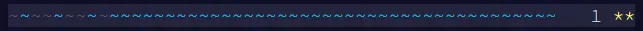Like in the past, I’m participating in this years https://adventofcode.com.

First of let’s look at the template that can be used for each day:

``````// See https://aka.ms/new-console-template for more information
Console.WriteLine("AoC 2021 Day 1");

.Select(line => int.Parse(line)).ToArray();

Console.WriteLine(\$"One: {PuzzleOne(input)}");
Console.WriteLine(\$"Two: {PuzzleTwo(input)}");

int PuzzleOne(int[] input)
{
throw new NotImplementedException();
}

int PuzzleTwo(int[] input)
{
throw new NotImplementedException();
}
``````

## Puzzle One

The idea of the code is to find a Linq expression which efficiently find’s pair’s – an element and it’s predecessor – which are compared.

I use the `Select()` 1 and `Sum()` 2 methods as follows:

``````int PuzzleOne(int[] input)
{
return input.Select((m, i) => (i != 0 && m > input[i - 1]) ? 1 : 0).Sum();
}
``````

The only trick is `(i != 0 && m > input[i - 1])` which prevents an out of bounds array access.

## Puzzle Two

If you read the puzzle description carefully, you’ll see that the computation of the sum is done in the same way as in the first puzzle. The difference is in the numbers to be added. So I simply define a function `GetWindows()` which generates the input for the function solving the first puzzle.

``````int PuzzleTwo(int[] input)
{
var windows = GetWindows(input);

return PuzzleOne(windows);
}

int[] GetWindows(int[] input)
{
return input.SkipLast(2).Select((m, i) => m + input[i + 1] + input[i + 2]).ToArray();
}
``````

The trick is to use `SkipLast()` 3, because the last elements of the input array aren’t used as start of a new window.

## Full Source

Full Source is available on GitHub. 4